# A Calculation Examples

This appendix contains the following sections:

## A.1 Getting More Information

For more information about Analytic Functions, and Oracle functions in general, refer to the following Oracle publications:

• Oracle Database SQL Reference

• Oracle Database Data Warehousing Guide

## A.2 About the examples in this chapter

The examples in the following sections use the Discoverer Desktop sample data.

## A.3 Additional formatting

The examples in this chapter use the following additional formatting:

• Currency is displayed with no decimal places, and is prefixed with a Dollar sign (\$).

• Integers are displayed with no decimal places. For example, Ranks.

## A.4 Simple Calculation Examples

This section provides the following examples to help you create Calculations.

### A.4.1 Calculate the number of rows returned by a query

This example calculates the number of rows returned by a query.

Workbook options Details
Select Items Video Analysis Information: Year (Calendar Year), Region, City, Sales SUM
Sort Order Year, Region, City
Conditions Department = Video Sale OR Department = Video Rental

Year = 2000 AND Region = Central

Calculation Name Rows returned
Calculation ROWCOUNT
Notes ROWCOUNT does not count NULL values. To calculate the number of rows returned by a query, including NULL values, first create a temporary item One record, (Calculation = '1'). Then, create a Calculation Rows returned to count the occurrences of One record, (SUM(Video Sales Analysis.One record))
Display Data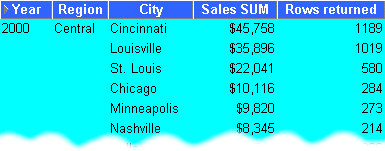### A.4.2 Calculate a 25% increase in sales

This example calculates a 25% increase in sales figures.

Workbook options Details
Select Items Video Analysis Information: Year (Calendar Year), Region, City, Sales SUM
Sort Order Year, Region, City
Conditions Department = Video Sale OR Department = Video Rental

Year = 2000 AND Region = Central

Calculation Name 25% Increase
Calculation Sales SUM * 1.25
Display Data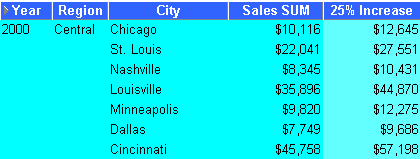### A.4.3 Convert text to upper-case

As well as the extensive range of mathematical functions available in Discoverer Desktop, you also have access to a wide range of number and text formatting functions. This example uses a Calculation to re-format City text data to upper-case.

Workbook options Details
Select Items Video Analysis Information: Year (Calendar Year), Region, City, Sales SUM
Sort Order Year, Region
Conditions Department = Video Sale OR Department = Video Rental

Year = 2000 AND Region = Central

Calculation Name City(Upper Case)
Calculation UPPER(City)
Display Data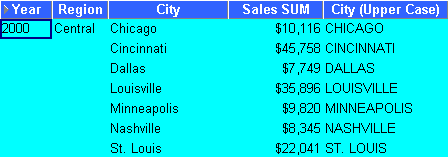## A.5 Analytic Function Examples

Oracle Business Intelligence Discoverer supports the Analytic Functions that are included in the Oracle database. Discoverer Desktop also extends the functionality of standard SQL by allowing you to nest Analytic Functions. You can use these advanced functions to perform sophisticated analysis on your data.

This section contains the following sub-sections:

### A.5.1 Analytic Function Categories

Analytic Functions are classified in the following categories:

• Ranking - Address business questions like: 'What are the top 10 and bottom 10 salespeople per region?'.

• Banding - Address business questions like 'What brands make up 25% of sales?'.

• Windowing - Address business questions like 'What is the 13-week moving average of a stock price?' or 'What is the cumulative sum of sales per region?'.

• Reporting Aggregates - After a query has been processed, aggregate values like the number of resulting rows, or the sum of a column in a set of rows. Address questions like 'What are each product's Sales as a percentage of Sales for its product group?'.

• Lag/Lead - Address business questions like 'Which regions growth of sales of 1998 as compared to 1997 was greater than 20%?', and 'What is the value of Sales in Financial Year 1996 minus Sales in Financial Year 1996?'.

• Statistics - Perform statistical analysis with Business Intelligence OLAP/spreadsheet applications. For example, covariance and linear regression functions.

### A.5.2 Calculations and Drilling Into and out of data

When you use Analytic Functions, note that they have a precise definition which does not change as you drill, pivot, or sort the result set. For example, if you use the RANK function to assign ranks to sales figures partitioned by Quarter, if you drill down the Month level, the rank still only applies to the Quarter level.

### A.5.3 About The Analytic Function Template

When creating Analytic Functions in Discoverer Desktop, you can either type or paste them directly into the Calculation dialog box, or you can select them from the function list.

If you select them from the function list, you are presented with a generic Analytic Function Template that helps you define the function by telling you what information you may need to provide. Templates should be used as a guide. Because templates are designed to cover most types of usage, you will not always need to use every part of the template.

For example, when you paste a new RANK Analytic Function into a calculation box, Discoverer Desktop provides the following template:

OVER (PARTITION BY expr1 ORDER BY expr2)

Although you can define a complex function using both expressions (expr1 and expr2), you can often define a simple function using only the ORDER BY expression; for example:

RANK()OVER(ORDER BY 'Sales')

This example ranks sales figures (defined in the 'Sales' item).

NOTE: By default, results data is sorted in ascending order (ASC), nulls first (NULLS FIRST).

For more information about expressions used by the Analytic Function template, refer to "More about the Analytic Function Template".

### A.5.4 Ranking Function Examples

#### A.5.4.1 About Ranking

Ranking functions compute the rank of an item with respect to other items in an ordered list.

#### A.5.4.2 Assign Ranks to sales figures

This example ranks to a set of sales figures.

Workbook options Details
Select Items Video Analysis Information: Year (Calendar Year), Region, City, Sales SUM
Sort Order Year, Region
Conditions Department = Video Sale OR Department = Video Rental

Year = 2000 AND Region = Central

Calculation Name Rank
Calculation RANK() OVER(ORDER BY Sales SUM DESC)
Notes By default, ranked results data is sorted in ascending order (ASC), nulls first (NULLS FIRST). The additional DESC parameter sorts the results in descending order, which ranks the highest value with the Rank 1.
Display Data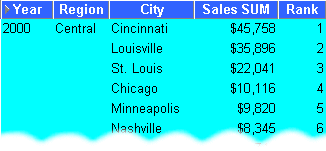#### A.5.4.3 Assign Ranks to sales figures within Region

This example ranks a set of sales figures within each Region for each Year.

Workbook options Details
Select Items Video Analysis Information: Year (Calendar Year), Region, City, Sales SUM
Sort Order Year, Region
Conditions Department = Video Sale OR Department = Video Rental

Year = 2000

Calculation Name Rank Top
Calculation RANK() OVER(PARTITION BY Year, Region ORDER BY Sales SUM DESC)
Display Data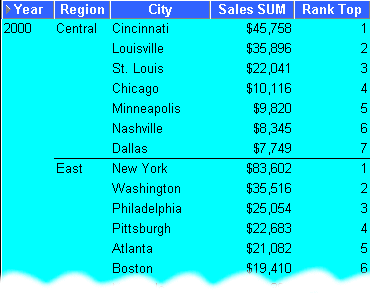#### A.5.4.4 Show the top three selling Cities per Region

This example ranks a set of sales figures and displays the top three selling Cities for each Region.

Workbook options Details
Select Items Video Analysis Information: Year (Calendar Year), Region, City, Sales SUM
Sort Order Year, Region
Conditions Department = Video Sale OR Department = Video Rental

Year = 2000

Rank Top <= 3

Calculation Name Rank Top
Calculation RANK() OVER(PARTITION BY Year, Region ORDER BY Sales SUM DESC)
Tip To quickly filter the list to the first, second, or third ranked cities, pivot the Rank Top item to the page axis, (see also "Pivoting Data on a Table").
Display Data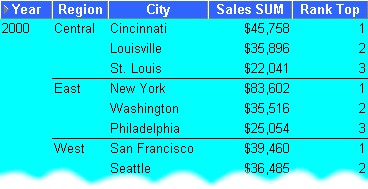#### A.5.4.5 Show the top three and bottom three selling Cities per Region

This example ranks a set of sales figures and displays the top three and bottom three performing Cities per Region.

Workbook options Details
Select Items Video Analysis Information: Year (Calendar Year), Region, City, Sales SUM
Sort Order Year, Region
Conditions Department = Video Sale OR Department = Video Rental

Year = 2000

Rank Top <= 3 OR Rank Bottom <= 3

Calculation Name Rank Top
Calculation RANK() OVER(PARTITION BY Year, Region ORDER BY Sales SUM DESC)
Additional Calculations Required Rank Bottom = RANK() OVER(PARTITION BY Year, Region ORDER BY Sales SUM ASC)
Notes This analysis involves three steps:

1 - Assign ranks to Cities on Sales SUM in descending order, as Rank Top.

2 - Assign ranks to Cities on Sales SUM in ascending order, as Rank Bottom.

3 - Displaying only Rank Top, filter the data using a Condition to return only the top three and bottom three ranked Brands.

In the example shown below, in the 'Central' Region, the top three cities are ranked 1, 2, and 3; the bottom three cities are ranked 5, 6, and 7. In the 'East' Region, the top three cities are ranked 1, 2, and 3; the bottom three cities are ranked 6, 7, and 8, and so on.

Display Data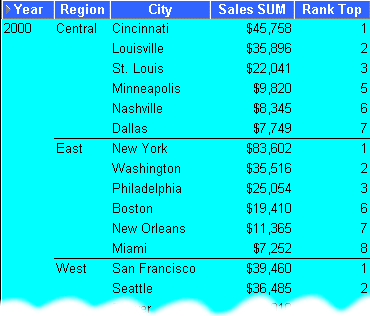### A.5.5 Banding Function Examples

#### A.5.5.1 About Banding

Banding is a type of ranking that divides a list of values in a partition into a specified number of groups called Bands (also known as buckets) and assigns each value to a Band.

Two common types of banding are:

• Banding by value - this divides values into groups according to their value, (also known as equi-width bands).

Here, the function typically takes the largest value minus the lowest value, and divides the result by the number of bands required. This value defines the range of each Band.

Values are then assigned to bands according to which range they fall into. Therefore, the number of values in each Band may differ. For example, if we have 100 values and divide them into four equi-width bands, each band may contain different numbers of values.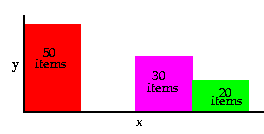Use the GREATEST function or the CASE function to produce equi-width bands based on value.

• Banding by rank - this divides values into groups according to their rank, (also known as equi-height bands).

Here, the function divides the number of values in the partition by the number of bands, which gives the number of values in each band.

An equal number of values are then placed in each band. For example, if we have 100 values and divide them into four equi-height bands, each band contains 25 values.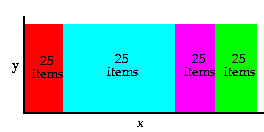Use the NTILE function to produce equi-height bands based on rank.

#### A.5.5.2 Producing equi-width Bands (1)

This example divides Sales figures into bands according to their value, (also known as equi-width bands).

Workbook options Details
Select Items Video Analysis Information: Year (Calendar Year), Region, City, Sales SUM
Sort Order Year, Region
Conditions Department = Video Sale OR Department = Video Rental

Year = 2000, Region = Central

Calculation Name Sales Bands
Calculation GREATEST(1,4-FLOOR((Sales SUM-Min Sales for Region)/GREATEST(1,FLOOR((Max Sales for Region-Min Sales for Region+1)/4))))
Additional Calculations Required Max Sales for Region = MAX(Sales SUM) OVER(PARTITION BY Region,Year)

Min Sales for Region = MIN(Sales SUM) OVER(PARTITION BY Region,Year)

Notes Using the Central Region and Year 2000 as an example, this function takes the largest value (45,758) minus the smallest value (7,749) and divides it by four, ((45,758-7,749)/4), giving four equal Bands of 9,502.25. This gives four bands with the following ranges:
• Band 1 - 36,255.75 to 45,758

• Band 2 - 26,753.5 to 36,255.75

• Band 3 - 17,251.25 to 26,753.5

• Band 4 - 7,749 to 17,251.25

Each value is placed in one of the four Bands depending on which range the Sales SUM value falls into.

Display Data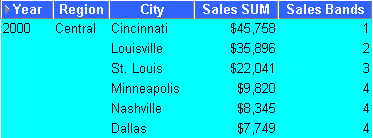#### A.5.5.3 Producing equi-width Bands (2)

This example creates the same results as the example in "Producing equi-width Bands (1)", except that it uses a CASE statement rather than the GREATEST function. Again, the example divides Sales figures into bands according to their value, using a CASE function, (see also "Producing equi-width Bands (1)").

Workbook options Details
Select Items Video Analysis Information: Year (Calendar Year), Region, City, Sales SUM
Sort Order Year, Region
Conditions Department = Video Sale OR Department = Video Rental

Year = 2000, Region = Central

Calculation Name Sales Bands 2
Calculation CASE WHEN Sales SUM < Q1 THEN 4 WHEN Sales SUM < Q2 THEN 3 WHEN Sales SUM < Q3 THEN 2 WHEN Sales SUM >= Q3 THEN 1 END
Additional Calculations Required MAX Sales = MAX(Sales SUM) OVER(PARTITION BY Year)

MIN Sales = MIN(Sales SUM) OVER(PARTITION BY Year)

Range = (MAX Sales - MIN Sales)/4

Q1 = MIN Sales + Range

Q2 = MIN Sales + (Range*2)

Q3 = MAX Sales - Range

Notes This function uses a series of IF statements in the form of a CASE function to assign sales figures into bands, (see Band Ranges below).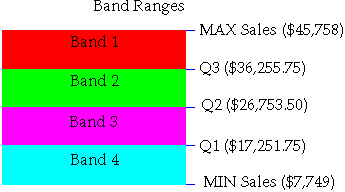Display Data#### A.5.5.4 Producing equi-height Bands

This example assigns a set of sales figures into two equi-height Bands.

Workbook options Details
Select Items Video Analysis Information: Year (Calendar Year), Region, City, Sales SUM
Sort Order Year, Region
Conditions Department = Video Sale OR Department = Video Rental

Year = 2000, Region = Central

Calculation Name Sales Bands 3
Calculation NTILE(2) OVER(PARTITION BY Year, Region ORDER BY Sales SUM DESC)
Notes Using the Central Region and Year 2000 as an example, this function takes the number of values (which is six) and divides it by two, giving three values per Band. It then takes the list of values ordered by Sales SUM and places values one, two, and three in Band 1, values four, five, and six in Band 2.
Display Data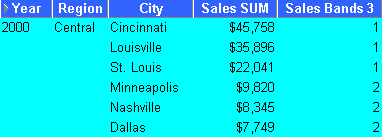### A.5.6 Windowing Function Examples

#### A.5.6.1 About Windowing

Windowing functions are used to compute aggregates using values from other rows. For example, cumulative, moving, and centered aggregates.

Two common types of windowing are:

• Windowing with logical offsets - here, the offset is based on a value relative to an existing value, e.g. three months preceding a date value.

For example, if we have a list of monthly sales figures, a logical window could compute a moving average of the previous three months, (inclusive of the current month).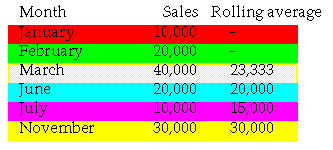When calculating the average, the calculation assumes a NULL value for months missing from the list. In the example, the three-month moving average for November assumes NULL values for the missing months September and October.

• Windowing with physical offsets - here, the offset is based on a value that is a specified number of rows from an existing value, e.g. three rows from the current item.

For example, if we have a list of monthly sales figures, a physical window could compute a moving average of the previous three rows ignoring missing months.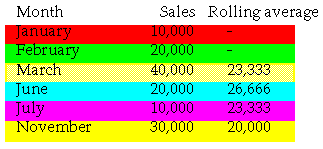When calculating the average, the calculation ignores months missing from the list. In the example, the three-month moving average for November uses June, July, and November in the calculation.

#### A.5.6.2 Calculate a three month moving sales average

This example uses a logical window to calculate a moving three month Sales average.

Workbook options Details
Select Items Video Analysis Information: Year (Calendar Year), Region, City, Sales SUM
Sort Order Year, Region
Conditions Department = Video Sale OR Department = Video Rental

Year = 2000, Region = Central

Calculation Name Sales Bands 3
Calculation NTILE(2) OVER(PARTITION BY Year, Region ORDER BY Sales SUM DESC)
Notes Using the Central Region and Year 2000 as an example, this function takes the number of values (which is six) and divides it by two, giving three values per Band. It then takes the list of values ordered by Sales SUM and places values one, two, and three in Band 1, values four, five, and six in Band 2.
Display Data#### A.5.6.3 Show the cumulative values of sales

This example uses a physical window to calculate the cumulative value of sales.

Workbook options Details
Select Items Video Analysis Information: Year (Calendar Year), Region, City, Sales SUM
Sort Order Year, Region
Conditions Department = Video Sale OR Department = Video Rental

Year = 2000, Region = Central

Calculation Name Cumulative Total
Calculation SUM(Sales SUM) OVER(ORDER BY Sales SUM DESC ROWS UNBOUNDED PRECEDING)
Display Data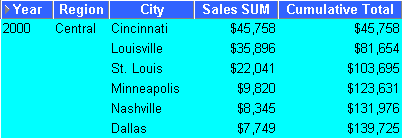### A.5.7 Reporting Function Examples

#### A.5.7.1 About Reporting Functions

Reporting functions are used to compute aggregates.

#### A.5.7.2 Calculate annual sales by Year

This example calculates annual sales by Year.

Workbook options Details
Select Items Video Analysis Information: Year (Calendar Year), Region, City, Sales SUM
Sort Order Year, Region
Conditions Department = Video Sale OR Department = Video Rental

Year = 2000

Calculation Name Annual Sales
Calculation SUM(Sales SUM) OVER()
Display Data#### A.5.7.3 Calculate annual sales by region

This example calculates the total annual sales by Year and Region.

Workbook options Details
Select Items Video Analysis Information: Year (Calendar Year), Region, City, Sales SUM
Sort Order Year, Region
Conditions Department = Video Sale OR Department = Video Rental

Year = 2000

Calculation Name Annual Sales by Region
Calculation SUM(Sales SUM) OVER(PARTITION BY Year, Region ORDER BY Year, Region)
Display Data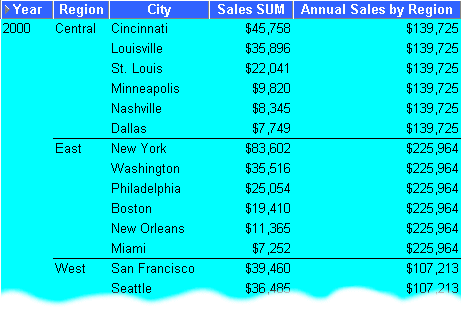#### A.5.7.4 Calculate percentage of annual sales by Region

This example calculates the percentage of annual sales per Region for each City in each Year.

Workbook options Details
Select Items Video Analysis Information: Year (Calendar Year), Region, City, Sales SUM
Sort Order Year, Region, % of Annual Sales
Conditions Department = Video Sale OR Department = Video Rental

Year = 2000

Calculation Name % of Annual Sales
Calculation Sales SUM*100/Annual Sales by Region
Additional Calculations Required Annual Sales by Region= SUM(Sales SUM) OVER(PARTITION BY Year, Region ORDER BY Year, Region)
Display Data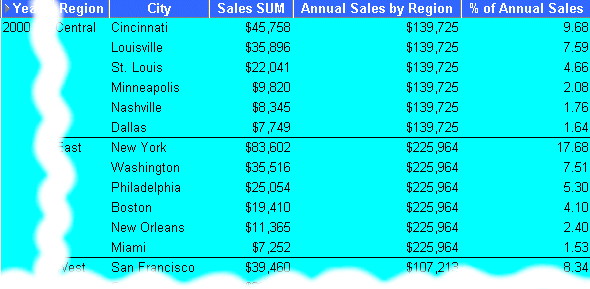#### A.5.7.5 Calculate sales as a percentage of total Sales

This example calculates sales as a percentage of total Sales.

Workbook options Details
Select Items Video Analysis Information: Year (Calendar Year), Region, City, Sales SUM
Sort Order Year, Region, % of total Sales
Conditions Department = Video Sale OR Department = Video Rental

Year = 2000, Region = Central

Calculation Name % of total Sales
Calculation RATIO_TO_REPORT(Sales SUM) OVER()*100
Display Data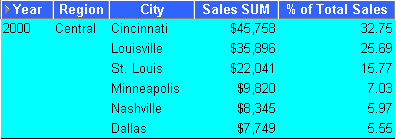### A.5.8 Lag/Lead Function Examples

#### A.5.8.1 About Lag/Lead Functions

LAG and LEAD functions are typically used to compare values in different time periods. For example, compare sales figures in 2000 with sales figures in 2001.

• LAG - provides access to more than one row of a table at the same time without a self-join.

• LEAD - provides access to a row at a given offset after the current position.

#### A.5.8.2 Compare sales figures across time

This example compares sales figures across time.

Workbook options Details
Select Items Video Analysis Information: Year (Calendar Year), Sales SUM
Sort Order Year
Conditions Department = Video Sale OR Department = Video Rental

Region = Central

Calculation Name Previous Year
Calculation LAG(Sales SUM,1) OVER(ORDER BY Year)
Notes Because there are no comparative figures for 1998, the Previous Year value for 1998 is blank.
Display Data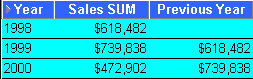#### A.5.8.3 Calculate sales growth across time

Using the comparative sales figures from example 11.8.2, this example calculates the sales growth across time.

Workbook options Details
Select Items Video Analysis Information: Year (Calendar Year), Sales SUM
Sort Order Year
Conditions Department = Video Sale OR Department = Video Rental

Region = Central

Calculation Name Growth
Calculation (Sales SUM-Previous Year)*100/Previous Year
Additional Calculations Required Previous Year = LAG(Sales SUM,1) OVER(ORDER BY Year)
Notes Because there are no comparative figures for 1998, the Previous Year value for 1998 is blank.
Display Data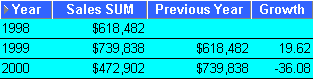#### A.5.8.4 Rank sales growth

Using the comparative sales figures from examples A.2.8.2 and A.2.8.3, this example ranks sales growth by year.

Workbook options Details
Select Items Video Analysis Information: Year (Calendar Year), Sales SUM
Sort Order Year
Conditions Department = Video Sale OR Department = Video Rental

Region = Central

Calculation Name Rank Growth
Calculation RANK() OVER(ORDER BY Growth DESC)
Additional Calculations Required Previous Year = LAG(Sales SUM,1) OVER(PARTITION BY 'Year' ORDER BY 'Year' )

Growth = (Sales SUM-Previous Year)*100/Previous Year

Notes Because there are no comparative figures for 1998, the Previous Year and Growth values for 1998 are blank and the Rank Growth is calculated as '1'.
Display Data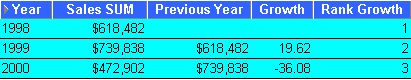### A.5.9 Statistical Function Examples

#### A.5.9.1 About Statistics Functions

Statistics functions are used to compute covariance, correlation, and linear regression statistics. Each function operates on an unordered set. They also can be used as windowing and reporting functions.

#### A.5.9.2 Calculate linear regression

This example computes an ordinary least-squares regression line that expresses the Profit SUM per Month as a linear function of its Sales SUM. The following functions are used:

• SLOPE - slope of determination of the regression line

• INTERCEPT - intercept of determination of the regression line

• REGR_R2 - coefficient of determination of the regression line

• REGR_COUNT - number of items

• REGR_AVGX - average salary

• REGR_AVGY - average bonus

Workbook options Details
Select Items Video Analysis Information: Year (Calendar Year), Month (Calendar Month), Sales SUM, Profit SUM
Sort Order Year
Conditions Department = Video Sale OR Department = Video Rental

Year = 2000

Calculations Slope = REGR_SLOPE(Profit SUM,Sales SUM) OVER(ORDER BY Profit SUM)

Intercept = REGR_INTERCEPT(Profit SUM,Sales SUM) OVER(ORDER BY Profit SUM)

Coefficient = REGR_R2(Profit SUM,Sales SUM) OVER(ORDER BY Profit SUM)

Count = REGR_COUNT(Profit SUM,Sales SUM) OVER(ORDER BY Profit SUM)

Average = REGR_AVGX(Profit SUM,Sales SUM) OVER(ORDER BY Profit SUM)

Average 2 = REGR_AVGY(Profit SUM,Sales SUM) OVER(ORDER BY Profit SUM)

Display Data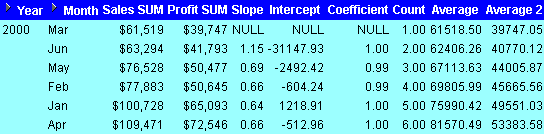## A.6 More about the Analytic Function Template

When you paste a new Analytic Function into a Calculation box, Discoverer Desktop provides the following generic template:

OVER (PARTITION BY expr1 ORDER BY expr2)

The expressions are used as follows.

• OVER - indicates that the function operates on a query result set, after the other query clauses have been applied, (such as FROM, WHERE, HAVING etc.

• PARTITION BY - partition (or group) the query results set. E.g. PARTITION BY 'Region'.

• ORDER BY - specify how the results set is logically ordered. E.g. ORDER BY 'Sales SUM'.

For more information about Oracle expressions, refer to "Getting More Information".

## A.7 Analytic Functions and Sequencing

When you use Analytic Functions in Conditions, the way that you combine them with non-Analytic Functions affects the data returned by the query. The following sequencing rules apply:

• Where Conditions contain only non-Analytic functions, these are applied before Conditions that contain Analytic functions.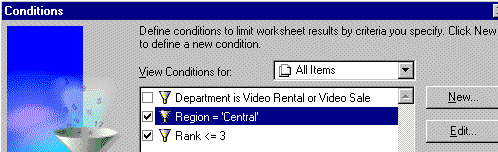In the example above, the 'Region = 'Central' condition is applied first, then the Rank is computed, then the 'Rank <= 3' condition is applied, (which contains an Analytic function).

• Where Conditions contain a combination of non-Analytic functions and Analytic functions, the Analytic functions are applied before the non-Analytic functions.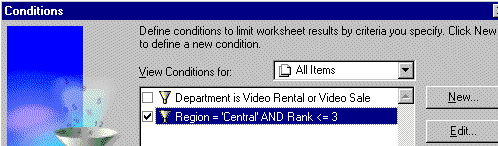In the example above, the Rank is evaluated, then the 'Rank <= 3' condition is applied, then the 'Region = 'Central' condition is applied.

To illustrate how sequencing affects the data returned by a query, consider the following two scenarios.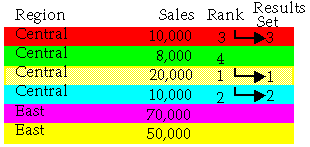In the first scenario above, we apply two simple Conditions: Region = 'Central', and Rank <= 3 (where Rank is an Analytic Function). The Region = 'Central' condition is applied first, then Rank <= 3. Therefore, only Sales figures for the Central Region that have a ranking of three or less are included in the Results Set.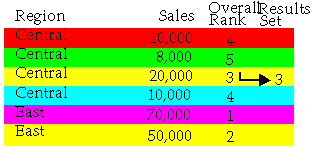In the second scenario above, we apply a single advanced Condition: Region = 'Central' AND Rank <= 3, (where Rank is an Analytic Function). The Rank <= 3 condition is applied first, then the Region = 'Central' condition. Therefore, only figures in the Central Region that have an overall ranking of three or less are included in the Results Set.